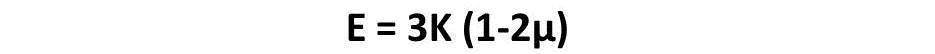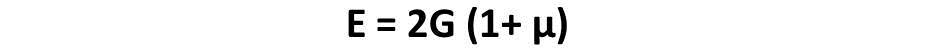# Relation between Young Modulus, Bulk Modulus, Modulus of Rigidity and Poisson’s Ratio

Sharing is Caring :)-

Today we will learn about relation between Young Modulus, Bulk Modulus and Modulus of Rigidity. All of these are elastic constant which are used to design any machinery part or structure. We have discussed about these three constant in our last post and know all of them are ratio of stress to strain in different conditions. Each elastic constant can be represent by two or more of these constant.

Let’s try to find out these relations.

### Relation between Young Modulus and Bulk Modulus:Where:

E = Young Modulus of Elasticity

K = Bulk Modulus of Elasticity

μ = Poisson’s Ratio

### Relation between Young Modulus and Modulus of Rigidity:Where:

E = Young Modulus of Elasticity

G = Modulus of Rigidity

μ = Poisson’s Ratio

### Relation between Bulk Modulus and Modulus of Rigidity:Where:

K = Bulk Modulus

G = Modulus of Rigidity

μ = Poisson’s Ratio

### Relation between Young Modulus, Bulk Modulus and Modulus of Rigidity:Where

E = Young Modulus of Elasticity

G = Modulus of Rigidity

K = Bulk Modulus

These are all most useful relations between all elastic constant which are used to solve any engineering problem related to them. If you have any query regarding or if you need any other information related to elastic constant, ask by commenting. If you like this article, don’t forget to share it on social networks. Subscribe our website for more informative articles. Thanks for reading it.

Sharing is Caring :)-

### One thought on “Relation between Young Modulus, Bulk Modulus, Modulus of Rigidity and Poisson’s Ratio”

•January 4, 2019 at 5:46 pm